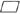## Special Quadrilaterals

Unlike humans, all quadrilaterals are not created equal. It's not a matter of size I'm alluding to here, but rather a question of features. They may have a pair of parallel sides, two pairs, a right angle ….

## Trapezoid

trapezoid is a quadrilateral with only one pair of opposite sides parallel. The parallel sides are called bases, and the nonparallel sides are called legs. A segment that joins the midpoints of the legs is called the median of the trapezoid. Any segment that is perpendicular to both bases is called an altitude of the trapezoid (Figure 1). The length of an altitude is called the height of the trapezoid.Figure 1 A trapezoid with its median and an altitude.

AB and CD are bases.

XY is an altitude.

MN is the median.

XY, length of segment XY is the height.

## Parallelogram

parallelogram is any quadrilateral with both pairs of opposite sides parallel. Each pair of parallel sides is called a pair of bases of the parallelogram. Any perpendicular segment between a pair of bases is called the an altitude of the parallelogram. The length of an altitude is the height of the parallelogram. The symbolis used for the word parallelogram. Figure shows that a parallelogram has two sets of bases and that, with each set of bases, there is an associated height.Figure 2 A parallelogram with its bases and associated heights.

InABCD,

XY is an altitude to bases AB and CD

JK is an altitude to bases AD and BC

XY is the height ofABCD, with AB and CD as bases, JK is the height ofABCD, with AD and BC as bases.

The following are theorems regarding parallelograms:

Theorem 41: A diagonal of a parallelogram divides it into two congruent triangles.

InABCD with diagonal BD according to Theorem 41, ΔABD ≅ Δ CDB (Figure 3).Figure 3 Two congruent triangles created by a diagonal of a parallelogram.

Theorem 42: Opposite sides of a parallelogram are congruent.

Theorem 43: Opposite angles of a parallelogram are congruent.

Theorem 44: Consecutive angles of a parallelogram are supplementary.

InABCD (Figure 4:Figure 4 A parallelogram.

• By Theorem 42, AB = DC and AD = BC.
• By Theorem 43, m ∠ A = m ∠ C and m ∠ B = m ∠ D.
• By Theorem 44:
• ∠ A and ∠ B are supplementary.
• ∠ B and ∠ C are supplementary.
• ∠ C and ∠ D are supplementary.
• ∠ A and ∠D are supplementary.

Theorem 45: The diagonals of a parallelogram bisect each other.

InABCD (Figure 5), by Theorem 45, AE = EC and BE = ED.Figure 5 The diagonals of a parallelogram bisect one another.

Back to Top
A18ACD436D5A3997E3DA2573E3FD792A